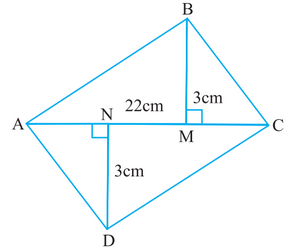# Q. 11.     Find the area of the quadrilateral . Here,  andR Riya

Area  of  quadrilateral ABCD = Area of triangle ABC + Area of tringle ADC

Area of triangle ABC with base 22 cm and height 3 cm is

Area of triangle ADX with base 22 cm and height 3 cm is

Therefore, the area of quadrilateral ABCD is =

Exams
Articles
Questions